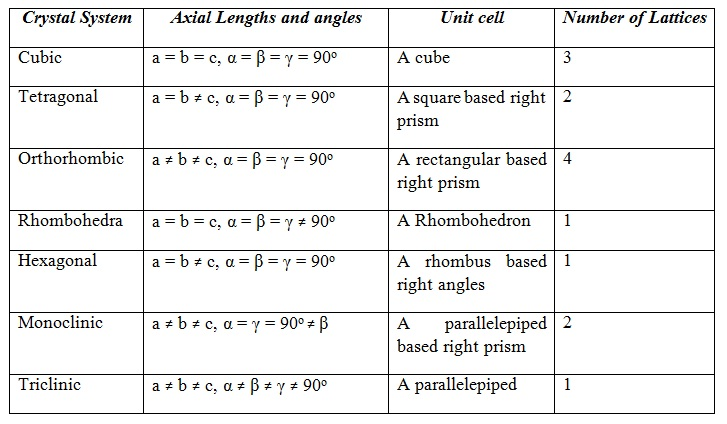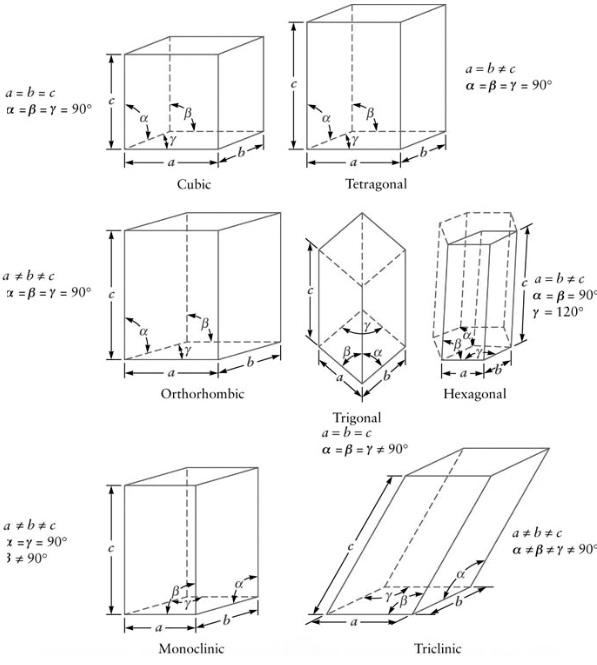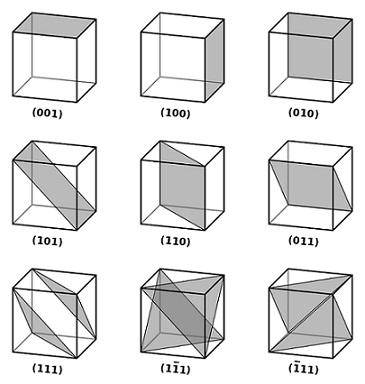#### Crystal classification, Physics tutorial

Introduction:

The Crystal lattices are categorized according to their symmetry properties, like inversion, reflection and rotation. As well, it is at times more convenient to deal with the non-primitive or conventional cells that have additional lattice sites either within the cell or on its surface. In three dimensions there are fourteen (14) different Bravais crystal lattices that belong to seven (7) crystal systems. Such systems are triclinic, monoclinic, orthorhombic, tetragonal, cubic, hexagonal and trigonal.

Definition of Crystal Lattice:

The Crystal lattice categorization is the regular geometric arrangement of points in the atom of a crystal.

Fundamental types of lattices:

The most apparent characteristic of a crystal is its symmetry or regularity. The basis of categorization of crystal is the symmetry represented by them. In a well stated crystal, the different symmetry elements (that is, rotation, reflection, inversion and so on) intersect at a point. Each and every set of symmetry elements intersecting at a point (that is, the centre of unit cell) is termed as a point-group. As there are 32 point groups, there are equivalent numbers of crystal classes that can be grouped altogether into seven groups termed as crystal systems. The table shown below comprises of the list explaining the different systems. The figure shown below shows represents seven crystal systems can be obtained through successive distortion of the cube.Direction indices:

To determine the direction indices, the given rules are employed:

1) Determining any vector in the desired direction.

2) Deduce this vector in terms of the basis (a, b, c).

3) Divide the coefficient of (a, b, c) by their greatest common divisor.

The resulting set of three integers u, v, w generally included in parentheses [uvw] states a direction. <uvw> signifies that all the vectors are equivalent to [uvw]. Negative sign in any of the numbers are pointed out by putting a bar over the number (u‾) Let a = 2, b = 3, c = 4 units and the vector be:

r = 6i + 12j + 10k

Then r = 3(2)i + 4(3)j + 2.5(4)k

Therefore, the coefficients of (a, b, c) are 3, 4, 2.5. The relevant greatest common divisor is 0.5. Therefore, the three numbers 6, 8, 5 are found. Therefore, for instance considered the indices of the direction are .

In the cubic system, u, v, w are proportional to the direction cosines of the selected vector. The cube edge 'a' would be represented by , that of direction 'b' by , and 'c' by .The negative direction of 'a' would be . Whenever we speak of  plane, we mean a plane parallel to  however cutting a axis at (1/2) a. The figure represents the indices of some significant planes and directions in crystals. Note that:

1) All parallel rows of atoms contain the same [uvw].

2) The angle θ between two crystallographic direction [u1v1w1] and [u2v2w2] in a cubic system is represented by:

Cos θ = (u1u2 + v1v2 + w1w2)/[u12 + v12 + w12)1/2 (u22 + v22 + w22)1/2]

Miller indices

The Miller indices are the most generally used notation for specifying the points, directions and planes in the crystal lattice systems.  Not only do they simplify the illustration of locations and directions within the lattice, however they as well allow vector operations such as dot and cross products. Miller Indices are the symbolic vector representation for the orientation of an atomic plane in the crystal lattice and are stated as the reciprocals of the fractional intercepts which the plane makes by the crystallographic axes. Prior to Miller indices can be employed; a coordinate system for the crystal structure should first be chosen.  The right-hand Cartesian coordinate system is the common choice for this. Points in the coordinate system are specified through Miller indices as h, k, l, here h, k, and l are the fractions of the lattice parameters a, b, and c.  Remember that a, b, and c are the lengths of the edges of the crystal's unit cell in the x, y and z directions.

Miller Indices are the symbolic vector representation for the orientation of an atomic plane in the crystal lattice and are stated as reciprocals of the fractional intercepts that plane makes by the crystallographic axes.

In an orthogonal coordinate system, the interplaner distance dhkl is represented by:

1/(dhkl)2 = (h/a)2 + (k/b)2 + (l/c)2

Here h, k, l are the Miller indices of the planes and a, b, c are dimensions of the unit cell.

Now for a cubic system, a = b =c

Therefore, dhkl = a/[h2 + k2 + l2]1/2

Determination of the Miller Indices:

The Rules for Miller Indices are:

- Find out the intercepts of the plane all along the three crystallographic axes, in terms of unit cell dimensions. The coordinates of the points of interception are deduced as integral multiples of the axial lengths in the respective directions. The integer p, q and r are the multiples of axial lengths a, b and c correspondingly

- Take the reciprocals of the integers p, q and r

- The reciprocals are decreased to the smallest set of integers h, k and l via taking LCM

- The integers are written as (hkl) via enclosing in parenthesis

For illustration, whenever the x-, y-, and z- intercepts are 2, 1, and 3, the Miller indices are computed as:

• The integers are 2, 1, 3
• Take reciprocals: 1/2, 1/1, 1/3
• Clear fractions (multiply by 6): 3, 6, 2
• Decrease to the lowest terms (already there)

Therefore, the Miller indices are 3, 6, 2. If a plane is parallel to the axis, its intercept is at infinity and its Miller index is zero. A generic Miller index is represented by (hkl). If a plane consists of negative intercept, the negative number is represented by a bar above the number. Never modify the negative numbers. For illustration, do not divide -1, -1, -1 by -1 to get 1, 1, 1. This implies symmetry that the crystal might not have!

General Principles of Miller Indices:

1) Whenever a Miller index is zero, then the plane is parallel to that axis.

2) The smaller a Miller index, the more almost parallel the plane is to the axis.

3) The larger a Miller index, the more nearly perpendicular a plane is to that axis.

4) Multiplying or dividing a Miller index through a constant has no effect on the orientation of the plane.

5) Miller indices are nearly always small.

The figure shown below represents a few planes for cubic lattices with their Miller notations.The Miller indices are stated as the reciprocals of the fractional intercepts which the plane forms by the crystallographic axes.

The angle θ between two crystallographic direction [u1v1w1] and [u2v2w2] in a cubic system is represented by:

Cos θ = (u1u2 + v1v2 + w1w2)/[u12 + v12 + w12)1/2 (u22 + v22 + w22)1/2]

The distance dhkl between neighboring planes of the family (hkl), is given in terms of the cube edge 'a' as:

dhkl = a/(h2 + k2 + l2)1/2

Tutorsglobe: A way to secure high grade in your curriculum (Online Tutoring)